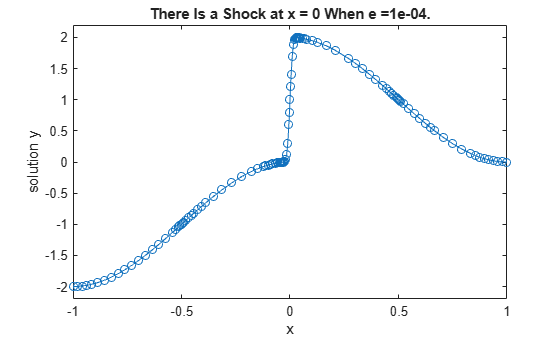Main Content

# Solve BVP Using Continuation

This example shows how to solve a numerically difficult boundary value problem using continuation, which effectively breaks the problem up into a sequence of simpler problems.

For $0<\mathit{e}\ll 1$, consider the differential equation

$\mathit{e}{{\mathit{y}}^{\prime }}^{\prime }+{\mathrm{xy}}^{\prime }=-\mathit{e}{\pi }^{2}\mathrm{cos}\left(\pi \mathit{x}\right)-\pi \mathrm{xsin}\left(\pi \mathit{x}\right)$.

The problem is posed on the interval $\left[-1,1\right]$ and is subject to the boundary conditions

$\mathit{y}\left(-1\right)=-2$,

$\mathit{y}\left(1\right)=0$.

When $\mathit{e}={10}^{-4}$, the solution to the equation undergoes a rapid transition near $\mathit{x}=0$, so it is difficult to solve numerically. Instead, this example uses continuation to iterate through several values of $\mathit{e}$ until $\mathit{e}={10}^{-4}$. The intermediate solutions are each used as the initial guess for the next problem.

To solve this system of equations in MATLAB, you need to code the equations, boundary conditions, and initial guess before calling the boundary value problem solver `bvp4c`. You either can include the required functions as local functions at the end of a file (as done here), or you can save them as separate, named files in a directory on the MATLAB path.

### Code Equation

Using the substitutions ${\mathit{y}}_{1}=\mathit{y}$ and ${\mathit{y}}_{2}={\mathit{y}}^{\prime }$, you can rewrite the equation as the system of first-order equations

${{\mathit{y}}_{1}}^{\prime }={\mathit{y}}_{2}$,

${{\mathit{y}}_{2}}^{\prime }=-\frac{\mathit{x}}{\mathit{e}}{\mathit{y}}^{\prime }-{\pi }^{2}\mathrm{cos}\left(\pi \mathit{x}\right)-\frac{\pi \mathit{x}}{\mathit{e}}\mathrm{sin}\left(\pi \mathit{x}\right)$.

Write a function to code the equations with the signature `dydx = shockode(x,y)`, where:

• `x` is the independent variable.

• `y` is the dependent variable.

• `dydx(1)` gives the equation for ${{\mathit{y}}_{1}}^{\prime }$, and `dydx(2)` gives the equation for ${{\mathit{y}}_{2}}^{\prime }$.

Make the function vectorized, so that `shockode([x1 x2 ...],[y1 y2 ...])` returns `[shockode(x1,y1) shockode(x2,y2) ...]`. This approach improves solver performance.

The corresponding function is

```function dydx = shockode(x,y) pix = pi*x; dydx = [y(2,:) -x/e.*y(2,:) - pi^2*cos(pix) - pix/e.*sin(pix)]; end ```

Note: All functions are included as local functions at the end of the example.

### Code Boundary Conditions

The BVP solver requires the boundary conditions to be in the form $\mathit{g}\left(\mathit{y}\left(\mathit{a}\right),\mathit{y}\left(\mathit{b}\right)\right)=0$. In this form the boundary conditions are:

$\mathit{y}\left(-1\right)+2=0$,

$\mathit{y}\left(1\right)=0$.

Write a function to code the boundary conditions with the signature `res = shockbc(ya,yb)`, where:

• `ya` is the value of the boundary condition at the beginning of the interval $\left[\mathit{a},\mathit{b}\right]$.

• `yb` is the value of the boundary condition at the end of the interval $\left[\mathit{a},\mathit{b}\right]$.

The corresponding function is

```function res = shockbc(ya,yb) % boundary conditions res = [ya(1)+2 yb(1)]; end ```

### Code Jacobians

The analytical Jacobians for the ODE function and boundary conditions can be calculated easily in this problem. Providing the Jacobians makes the solver more efficient, since the solver no longer needs to approximate them with finite differences.

For the ODE function, the Jacobian matrix is

${\mathit{J}}_{\mathrm{ODE}}=\frac{\partial \mathit{f}}{\partial \mathit{y}}=\left[\begin{array}{cc}\frac{\partial {\mathit{f}}_{1}}{\partial {\mathit{y}}_{1}}& \frac{\partial {\mathit{f}}_{1}}{\partial {\mathit{y}}_{2}}\\ \frac{\partial {\mathit{f}}_{2}}{\partial {\mathit{y}}_{1}}& \frac{\partial {\mathit{f}}_{2}}{\partial {\mathit{y}}_{2}}\end{array}\right]=\left[\begin{array}{cc}0& 1\\ 0& -\frac{\mathit{x}}{\mathit{e}}\end{array}\right]$.

The corresponding function is

```function jac = shockjac(x,y,e) jac = [0 1 0 -x/e]; end ```

Similarly, for the boundary conditions, the Jacobian matrices are

${\mathit{J}}_{\mathit{y}\left(\mathit{a}\right)}=\left[\begin{array}{cc}1& 0\\ 0& 0\end{array}\right]$ , ${\mathit{J}}_{\mathit{y}\left(\mathit{b}\right)}=\left[\begin{array}{cc}0& 0\\ 1& 0\end{array}\right]$.

The corresponding function is

```function [dBCdya,dBCdyb] = shockbcjac(ya,yb) dBCdya = [1 0; 0 0]; dBCdyb = [0 0; 1 0]; end ```

### Form Initial Guess

Use a constant guess for the solution on a mesh of five points in $\left[-1,1\right]$.

`sol = bvpinit([-1 -0.5 0 0.5 1],[1 0]);`

### Solve Equation

If you try to solve the equation directly using $\mathit{e}={10}^{-4}$, then the solver is not able to overcome the poor conditioning of the problem near the $\mathit{x}=0$ transition point. Instead, to obtain the solution for $\mathit{e}={10}^{-4}$, this example uses continuation by solving a sequence of problems for ${10}^{-2}$, ${10}^{-3}$, and ${10}^{-4}$. The output from the solver in each iteration acts as the guess for the solution in the next iteration (this is why the variable for the initial guess from `bvpinit` is `sol`, and the output from the solver is also named `sol`).

Since the value of the Jacobian depends on the value of $\mathit{e}$, set the options in the loop specifying the `shockjac` and `shockbcjac` functions for the Jacobians. Also, turn vectorization on since `shockode` is coded to handle vectors of values.

```e = 0.1; for i = 2:4 e = e/10; options = bvpset('FJacobian',@(x,y) shockjac(x,y,e),'BCJacobian',@shockbcjac,'Vectorized','on'); sol = bvp4c(@(x,y) shockode(x,y,e),@shockbc, sol, options); end```

### Plot Solution

Plot the results from `bvp4c` for the mesh $\mathit{x}$ and solution $\mathit{y}\left(\mathit{x}\right)$. With continuation, the solver is able to handle the discontinuity at $\mathit{x}=0$.

```plot(sol.x,sol.y(1,:),'-o'); axis([-1 1 -2.2 2.2]); title(['There Is a Shock at x = 0 When e =' sprintf('%.e',e) '.']); xlabel('x'); ylabel('solution y');```### Local Functions

Listed here are the local functions that the BVP solver `bvp4c` calls to calculate the solution. Alternatively, you can save these functions as their own files in a directory on the MATLAB path.

```function dydx = shockode(x,y,e) % equation to solve pix = pi*x; dydx = [y(2,:) -x/e.*y(2,:) - pi^2*cos(pix) - pix/e.*sin(pix)]; end %------------------------------------------- function res = shockbc(ya,yb) % boundary conditions res = [ya(1)+2 yb(1)]; end %------------------------------------------- function jac = shockjac(x,y,e) % jacobian of shockode jac = [0 1 0 -x/e]; end %------------------------------------------- function [dBCdya,dBCdyb] = shockbcjac(ya,yb) % jacobian of shockbc dBCdya = [1 0; 0 0]; dBCdyb = [0 0; 1 0]; end %-------------------------------------------```

Download ebook